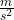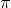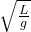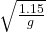Question

A simple pendulum is 1.15 meters long and has a period of 6.29 seconds. The pendulum is on an unknown planet. What is the acceleration of gravity of the Unknown Planet

1.thnahdat

The value of the acceleration of gravity of the Unknown Planet = 1.14Explanation:

length of the pendulum (L)= 1.15 m

Time period (T)= 6.29 seconds

We know that time period  of a simple pendulum is given by

⇒ T = 2×put the values in the above formula we get

⇒ T = 2×⇒ 6.29 = 2×By solving the above equation we get

g = 1.14This is the value of the acceleration of gravity of the Unknown Planet.